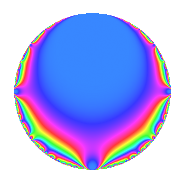Properties

 Label 23.1.bLevel 23 Weight 1 Character orbit b Rep. character $$\chi_{23}(22,\cdot)$$ Character field $$\Q$$ Dimension 1 Newforms 1 Sturm bound 2 Trace bound 0

Related objects

Defining parameters

 Level: $$N$$ = $$23$$ Weight: $$k$$ = $$1$$ Character orbit: $$[\chi]$$ = 23.b (of order $$2$$ and degree $$1$$) Character conductor: $$\operatorname{cond}(\chi)$$ = $$23$$ Character field: $$\Q$$ Newforms: $$1$$ Sturm bound: $$2$$ Trace bound: $$0$$

Dimensions

The following table gives the dimensions of various subspaces of $$M_{1}(23, [\chi])$$.

Total New Old
Modular forms 2 2 0
Cusp forms 1 1 0
Eisenstein series 1 1 0

The following table gives the dimensions of subspaces with specified projective image type.

$$D_n$$ $$A_4$$ $$S_4$$ $$A_5$$
Dimension 1 0 0 0

Trace form

 $$q$$ $$\mathstrut -\mathstrut q^{2}$$ $$\mathstrut -\mathstrut q^{3}$$ $$\mathstrut +\mathstrut q^{6}$$ $$\mathstrut +\mathstrut q^{8}$$ $$\mathstrut +\mathstrut O(q^{10})$$ $$q$$ $$\mathstrut -\mathstrut q^{2}$$ $$\mathstrut -\mathstrut q^{3}$$ $$\mathstrut +\mathstrut q^{6}$$ $$\mathstrut +\mathstrut q^{8}$$ $$\mathstrut -\mathstrut q^{13}$$ $$\mathstrut -\mathstrut q^{16}$$ $$\mathstrut +\mathstrut q^{23}$$ $$\mathstrut -\mathstrut q^{24}$$ $$\mathstrut +\mathstrut q^{25}$$ $$\mathstrut +\mathstrut q^{26}$$ $$\mathstrut +\mathstrut q^{27}$$ $$\mathstrut -\mathstrut q^{29}$$ $$\mathstrut -\mathstrut q^{31}$$ $$\mathstrut +\mathstrut q^{39}$$ $$\mathstrut -\mathstrut q^{41}$$ $$\mathstrut -\mathstrut q^{46}$$ $$\mathstrut -\mathstrut q^{47}$$ $$\mathstrut +\mathstrut q^{48}$$ $$\mathstrut +\mathstrut q^{49}$$ $$\mathstrut -\mathstrut q^{50}$$ $$\mathstrut -\mathstrut q^{54}$$ $$\mathstrut +\mathstrut q^{58}$$ $$\mathstrut +\mathstrut 2q^{59}$$ $$\mathstrut +\mathstrut q^{62}$$ $$\mathstrut +\mathstrut q^{64}$$ $$\mathstrut -\mathstrut q^{69}$$ $$\mathstrut -\mathstrut q^{71}$$ $$\mathstrut -\mathstrut q^{73}$$ $$\mathstrut -\mathstrut q^{75}$$ $$\mathstrut -\mathstrut q^{78}$$ $$\mathstrut -\mathstrut q^{81}$$ $$\mathstrut +\mathstrut q^{82}$$ $$\mathstrut +\mathstrut q^{87}$$ $$\mathstrut +\mathstrut q^{93}$$ $$\mathstrut +\mathstrut q^{94}$$ $$\mathstrut -\mathstrut q^{98}$$ $$\mathstrut +\mathstrut O(q^{100})$$

Decomposition of $$S_{1}^{\mathrm{new}}(23, [\chi])$$ into irreducible Hecke orbits

Label Dim. $$A$$ Field Image CM RM Traces $q$-expansion
$$a_2$$ $$a_3$$ $$a_5$$ $$a_7$$
23.1.b.a $$1$$ $$0.011$$ $$\Q$$ $$D_{3}$$ $$\Q(\sqrt{-23})$$ None $$-1$$ $$-1$$ $$0$$ $$0$$ $$q-q^{2}-q^{3}+q^{6}+q^{8}-q^{13}-q^{16}+\cdots$$